Next: Poisson's equation Up: The chaotic pendulum Previous: Periodic windows

## Further investigation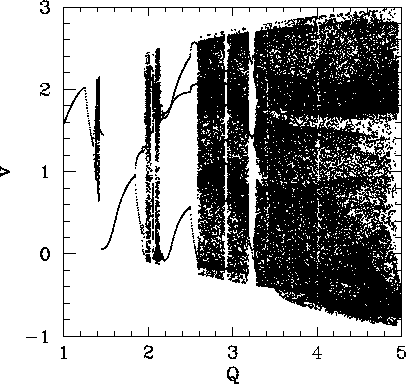Figure 58 shows the complete bifurcation diagram for the damped, periodically driven, pendulum (with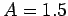and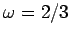). It can be seen that the chaotic region investigated in the previous section is, in fact, the first, and least extensive, of three different chaotic regions.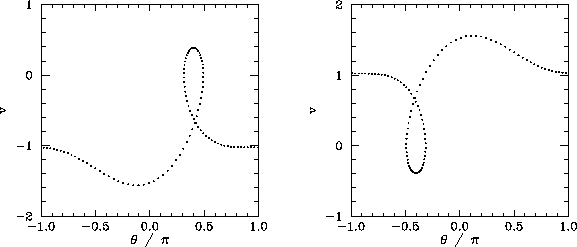The interval between the first and second chaotic regions is occupied by the period-1 orbits shown in Fig. 59. Note that these orbits differ somewhat from previously encountered period-1 orbits, because the pendulum executes a complete rotation (either to the left or to the right) every period of the external drive. Now, an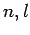periodic orbit is defined such that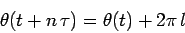for all(after the transients have died away). It follows that all of the periodic orbits which we encountered in previous sections were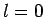orbits: i.e., their associated motions did not involve a net rotation of the pendulum. The orbits show in Fig. 59 are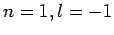and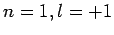orbits, respectively. The existence of periodic orbits in which the pendulum undergoes a net rotation, either to the left or to the right, is another example of spatial symmetry breaking--there is nothing in the pendulum's equations of motion which distinguishes between the two possible directions of rotation.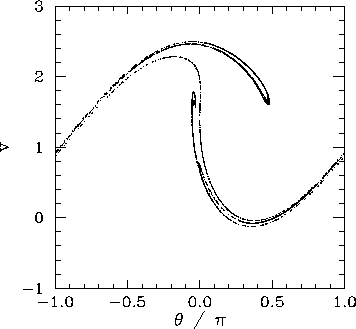Figure 60 shows the Poincaré section of a typical attractor in the second chaotic region shown in Fig. 58. It can be seen that this attractor is far more convoluted and extensive than the simple 4-line chaotic attractor pictured in Fig. 48. In fact, the attractor shown in Fig. 60 is clearly a fractal curve. It turns out that virtually all chaotic attractors exhibit fractal structure.

The interval between the second and third chaotic regions shown in Fig. 58 is occupied by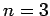,periodic orbits. Figure 61 shows the Poincaré section of a typical attractor in the third chaotic region. It can be seen that this attractor is even more overtly fractal in nature than that pictured in the previous figure. Note that the fractal nature of chaotic attractors is closely associated with some of their unusual properties. Trajectories on a chaotic attractor remain confined to a bounded region of phase-space, and yet they separate from their neighbours exponentially fast (at least, initially). How can trajectories diverge endlessly and still stay bounded? The basic mechanism is described below. If we imagine a blob of initial conditions in phase-space, then these undergo a series of repeated stretching and folding episodes, as the chaotic motion unfolds. The stretching is what gives rise to the divergence of neighbouring trajectories. The folding is what ensures that the trajectories remain bounded. The net result is a phase-space structure which looks a bit like filo dough--in other words, a fractal structure.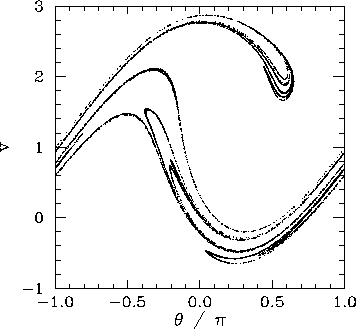Next: Poisson's equation Up: The chaotic pendulum Previous: Periodic windows
Richard Fitzpatrick 2006-03-29